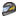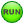# MV-8001: Path and Velocity Following

In this tutorial, you will learn how to define a path and velocity or acceleration profile, set up a feedforward steering controller to follow a path, and set up a feedforward traction controller to follow a velocity or acceleration profile.

Feedforward Controllers
In a feed-forward system, the control variable adjustment is not error-based. Instead it is based on knowledge about the process in the form of a mathematical model of the process and knowledge about or measurements of the process disturbances.
In simpler words, controller that uses the knowledge about the vehicle, to compute the signals.
Assume, a driver who knows that the vehicle weighs 1000 Kg and 30% throttle produces 1000 N of force in forward direction. If asked to produce 1 m/s2 of acceleration would simply give 30% throttle.
Defining a path for the driver
Multiple methods can be used to provide the desired path:
Table of centerline points
Path is provided as a table of equally spaced cartesian coordinates of centerline points. These points are provided in a separate file, DDF or Driver Demand File.
$Example DDF [ALTAIR_HEADER] FILE_TYPE = 'DDF' FILE_VERSION = 1.0 FILE_FORMAT = 'ASCII'$---------------------------------------------------UNITS
[UNITS]
(BASE)
{length  force      angle       mass    time}
'm'   'newton'   'degrees'   'kg'    'sec'
$---------------------------------DEMAND_VECTORS [DEMAND_VECTORS] {X Y Z} 0 0 0 -4 0 0 -1 0 0 -2 0 0 -5 0 0 -3 0 0 Sequence of straights and arcs Path is provided as a table of straights and circular section. KEY PAR0 PAR1 ST Length of the straight section Unused ARC Radius of curvature Angle of the arc • Positive angle means anti-clockwise arc • Negative angle means clockwise arc [PATH] {KEY PAR0 PAR1} 'ST' 100.0 0 'ARC' 50.0 1.57079 'ST' 100.0 0 'ARC' 50.0 3.14159 'ST' 200.0 0 'ARC' 50.0 -2.35619 Predefined path Path is one of the predefined paths visualization (Constant Radius, Single lane change, Double lane change, and Slalom). $Example block for constant radius path
[PATH]
INITIAL_STRAIGHT 	= 45
TURN 			= 'LEFT‘
Defining a velocity or acceleration profile for the driver
Demand velocity or acceleration profile is similar to open loop signal explained in tutorial 1. All methods – constant, expression and curve are valid for demand signal definition as well.

## Assemble the Vehicle

In this step, you will assemble the vehicle using the Assembly Wizard.

1. Start a new MotionView session.
2. Ensure the MBD-Vehicle Dynamics Tools preference file loaded is for all of the MotionView functionality of the Advanced Driver to work properly.
3. From the menu bar, select Model > Assembly Wizard.
In Script an Open Loop Acceleration Event, a linear torque map powertrain will be used to avoid the complexity of adding controllers for gear and clutch.
4. Select the Full vehicle with advanced driver option.
This takes care of all the dependencies of the advanced driver.
5. Click Next.
6. Choose the default selections in the following Assembly Wizard pages.
Page Label Selection Default (Yes/No)
1 Model type Full vehicle with driver No
2 Driveline configuration Front wheel drive Yes
3 Vehicle body Body Yes
3 Instrumentation Instrumentation Yes
3 Front subframe None Yes
3 Front suspension Frnt macpherson susp (1 pc. LCA) Yes
3 Steering linkages Rackpin steering Yes
3 Rear subframe None Yes
3 Powertrain Linear torque map powertrain Yes
3 Signal generator Driver signal generator Yes
4 Tires Auto Tires Yes
4 Steering column Steering column 1 (not for Abaqus) Yes
4 Steering boost None Yes
5 Front struts Frnt strut (with inline jts) Yes
5 Front stabilizer bars None Yes
5 Rear struts Rear strut (with inline jts) Yes
5 Rear stabilizer bars None Yes
6 Front jounce bumpers None Yes
6 Front rebound bumpers None Yes
6 Rear jounce bumpers None Yes
6 Rear rebound bumpers None Yes
7 Disk brakes Disk brakes Yes
7 Front driveline Independent fwd Yes
8 Driver System Altair Driver Yes
8   Next No
9   Finish No

In this step, you will use the Task Wizard to load the driver analysis.

2. Select AltairDriverFile from the Type drop-down menu.
Tip:

Selecting(Altair Driver icon) will open up the Altair Driver panel. This automatically resolves all of the vehicle attachments for the Altair Driver.

Selecting the AltairDriverFile 0 will open up the driver event panel. Selecting the Event Editor button will open the Altair Driver File dialog.

## Specify Vehicle Parameters

In this step, you will specify parameters for the vehicle.

Feedforward controllers require vehicle parameters because they model the vehicle. Vehicle parameters do not need to be precise. Most of the vehicle parameters required by the driver can be automatically calculated from the vehicle model.
Specify the parameters for the vehicle.

## Write an Altair Driver File Driving Event

In this step, you will create a constant radius with constant velocity event, a straight line acceleration event, and a path as a table of cartesian coordinates of centerline points event.

Driver requires an event script or the Altair driver file (ADF) to run any driving event. ADF can be edited using any text editor or by clicking the Edit Driver File button on the driver panel.

## Constant Radius with Constant Velocity Event

1. Open any text editor and copy and paste the following text into it.
Important: All blank lines must be removed prior to saving the file.
$-----------------------------------------------------------------ALTAIR_HEADER$ This block is required for version control
FILE_VERSION 	= 1.0
FILE_FORMAT 	= 'ASCII'
$--------------------------------------------------------------------------UNITS$In this block we specify the units in which this file should be read
[UNITS]
(BASE)
{length  force      	angle       mass   time}
$--------------------------------------------------------------------VEHICLE_IC$In this block we specify the initial conditions specifically initial speed of the
$vehicle with respect to the vehicle IC marker in the driver attachments [VEHICLE_INITIAL_CONDITIONS] VX0 = -15.0 VY0 = 0.0 VZ0 = 0.0$--------------------------------------------------------------STEERING_STANDARD
$This block specifies the saturation and cutoff frequency for the low pass filter for$steering output signal. These signals are global and are active for the entire event
[STEER_STANDARD]
MAX_VALUE 			= 3.141593
MIN_VALUE 			= -3.141593
SMOOTHING_FREQUENCY 	= 10.0
INITIAL_VALUE 		= 0.0
$--------------------------------------------------------------THROTTLE_STANDARD$This block specifies the saturation and cutoff frequency for the low pass filter for
$throttle output signal [THROTTLE_STANDARD] MAX_VALUE = 1.0 MIN_VALUE = 0.00 SMOOTHING_FREQUENCY = 10.0 INITIAL_VALUE = 0.5$---------------------------------------------------------------BRAKING_STANDARD
$This block specifies the saturation and cutoff frequency for the low pass filter for$brake output signal
[BRAKE_STANDARD]
MAX_VALUE 			= 1.0
MIN_VALUE 			= 0.0
SMOOTHING_FREQUENCY 	= 10.0
INITIAL_VALUE 		= 0.0
$-----------------------------------------------------------------MANEUVERS_LIST$This block provides the list of all the maneuvers, simulation time for each maneuver
$maximum solver step size (hmax) and print interval [MANEUVERS_LIST] { name simulation_time h_max print_interval} 'MANEUVER_1' 15.0 0.005 0.01$---------------------------------------------------------------------MANEUVER_1
[MANEUVER_1]
$This block provides the ties controllers to each driver output TASK = 'STANDARD' (CONTROLLERS) {DRIVER_SIGNAL PRIMARY_CONTROLLER ADDITIONAL_CONTROLLER} STEER FEEDFORWARD_STEER NONE THROTTLE FEEDFORWARD_TRACTION NONE BRAKE FEEDFORWARD_TRACTION NONE$---------------------------------------------------------STEER
$This is controller block containing all the information required by$the driver to construct the controller. Different controllers have
$different requirements. Here we are using feedforward steering$controller.
[FEEDFORWARD_STEER]
TAG 			= 'FEEDFORWARD'
PATH 			= 'PREDEFINED'
BLOCK 		= 'PATH'
$---------------------------------------------------------PATH$Block containing the information about the path to be followed
[PATH]
INITIAL_STRAIGHT 	= 20
TURN 			= 'LEFT'
$--------------------------------------------------THROTTLE & BRAKE [FEEDFORWARD_TRACTION] TAG = 'FEEDFORWARD' TYPE = 'FOLLOW_VELOCITY' LOOK_AHEAD_TIME = 0.5 DEMAND_SIGNAL = 'DEMAND_SPEED'$---------------------------------------------------------DEMAND_SPEED
$Block containing all the information about the velocity profile to be followed [DEMAND_SPEED] TYPE = 'CONSTANT' VALUE = 15.0 2. Save the ADF. 3. Clickto run the simulation. 4. Observe the results. 5. Complete the same path using a sequence of straight and arcs method. This is simply a change in the method of providing the same path and should not affect the results. 1. Change the PATH attribute in the FEEDFORWARD_STEER block in the ADF to SEQUENCE_OF_ST_CRV. [FEEDFORWARD_STEER] TAG = 'FEEDFORWARD' LOOK_AHEAD_TIME = 0.5 PATH = 'SEQUENCE_OF_ST_CRV ' BLOCK = 'PATH' 2. Replace the path block in the ADF with the following text. [PATH] {KEY PAR0 PAR1} 'ST' 20 0 'ARC' 40 6.28318 3. Rerun the simulation. The new results should match the initial results. ## Straight Line Acceleration Event In this step, you will create an event to follow a straight line while accelerating the vehicle constantly with 2 m/s2. 1. Open any text editor and copy and paste the following text into it. Important: All blank lines must be removed prior to saving the file. $-----------------------------------------------------------------ALTAIR_HEADER
FILE_VERSION 	= 1.0
FILE_FORMAT 	= 'ASCII'
$--------------------------------------------------------------------------UNITS [UNITS] (BASE) {length force angle mass time} 'meter' 'newton' 'radians' 'kg' 'sec'$--------------------------------------------------------------------VEHICLE_IC
[VEHICLE_INITIAL_CONDITIONS]
VX0 = -20.0
VY0 = 0.0
VZ0 = 0.0
$--------------------------------------------------------------STEERING_STANDARD [STEER_STANDARD] MAX_VALUE = 3.141593 MIN_VALUE = -3.141593 SMOOTHING_FREQUENCY = 10.0 INITIAL_VALUE = 0.0$--------------------------------------------------------------THROTTLE_STANDARD
[THROTTLE_STANDARD]
MAX_VALUE 			= 1.0
MIN_VALUE 			= 0.00
SMOOTHING_FREQUENCY 	= 10.0
INITIAL_VALUE 			= 0.2
$---------------------------------------------------------------BRAKING_STANDARD [BRAKE_STANDARD] MAX_VALUE = 1.0 MIN_VALUE = 0.0 SMOOTHING_FREQUENCY = 10.0 INITIAL_VALUE = 0.0$-----------------------------------------------------------------MANEUVERS_LIST
[MANEUVERS_LIST]
{ name       		simulation_time  h_max  print_interval}
'MANEUVER_1'		10.0                     0.01     0.01
$---------------------------------------------------------------------MANEUVER_1 [MANEUVER_1]$This block provides the ties controllers to each driver output
(CONTROLLERS)
STEER                   	FEEDFORWARD_STEER	  		NONE
THROTTLE             	FEEDFORWARD_TRACTION	  		NONE
BRAKE                   	FEEDFORWARD_TRACTION 			NONE
$---------------------------------------------------------STEER$This is controller block containing all the information required by
$the driver to construct the controller. Different controllers have$different requirements. Here we are using feedforward steering
$controller. [FEEDFORWARD_STEER] TAG = 'FEEDFORWARD' LOOK_AHEAD_TIME = 0.5 PATH = 'PREDEFINED' BLOCK = 'PATH'$---------------------------------------------------------PATH
$Block containing the information about the path to be followed [PATH] TYPE = 'CONSTANT_RADIUS' RADIUS = 40 INITIAL_STRAIGHT = 20 TURN = 'LEFT'$--------------------------------------------------THROTTLE & BRAKE
[FEEDFORWARD_TRACTION]
TAG 			= 'FEEDFORWARD'
TYPE 			= 'FOLLOW_ACCELERATION'
DEMAND_SIGNAL 	= 'DEMAND_ACC'
$---------------------------------------------------------DEMAND_ACC$Block containing all the information about the acceleration profile to be followed
[DEMAND_SPEED]
TYPE 			= 'CONSTANT'
VALUE			= 2.0
2. Clickto run the simulation.
3. Observe the results.

## Path as a Table of Cartesian Coordinates of Centerline Points Event

In this step, you will give a path as a table of Cartesian coordinated of centerline points and define the velocity profile along the path.

1. Open text editor and copy and paste the following text into it.
Important: All blank lines must be removed prior to saving the file.
$-----------------------------------------------------------------ALTAIR_HEADER [ALTAIR_HEADER] FILE_TYPE = 'ADF' FILE_VERSION = 1.0 FILE_FORMAT = 'ASCII'$--------------------------------------------------------------------------UNITS
[UNITS]
(BASE)
{length  force      	angle       mass   time}
$--------------------------------------------------------------------VEHICLE_IC [VEHICLE_INITIAL_CONDITIONS] VX0 = -20.0 VY0 = 0.0 VZ0 = 0.0$--------------------------------------------------------------STEERING_STANDARD
[STEER_STANDARD]
MAX_VALUE 			= 3.141593
MIN_VALUE 			= -3.141593
SMOOTHING_FREQUENCY 	= 10.0
INITIAL_VALUE 		= 0.0
$--------------------------------------------------------------THROTTLE_STANDARD [THROTTLE_STANDARD] MAX_VALUE = 1.0 MIN_VALUE = 0.00 SMOOTHING_FREQUENCY = 10.0 INITIAL_VALUE = 0.2$---------------------------------------------------------------BRAKING_STANDARD
[BRAKE_STANDARD]
MAX_VALUE 			= 1.0
MIN_VALUE 			= 0.0
SMOOTHING_FREQUENCY 	= 10.0
INITIAL_VALUE 			= 0.0
$-----------------------------------------------------------------MANEUVERS_LIST [MANEUVERS_LIST] { name simulation_time h_max print_interval} 'MANEUVER_1' 10.0 0.01 0.01$---------------------------------------------------------------------MANEUVER_1
[MANEUVER_1]
(CONTROLLERS)
STEER                   	FEEDFORWARD_STEER	  		NONE
THROTTLE             	FEEDFORWARD_TRACTION	  		NONE
BRAKE                   	FEEDFORWARD_TRACTION 			NONE
$---------------------------------------------------------STEER [FEEDFORWARD_STEER] TAG = 'FEEDFORWARD' LOOK_AHEAD_TIME = 0.5$Instruction to the driver that the path is of type DDF
PATH 			= 'DDF'
$Path of the ddf file, data lies in same folder in file named snet.ddf FILE = 'snet.ddf'$--------------------------------------------------THROTTLE & BRAKE
[FEEDFORWARD_TRACTION]
TAG 			= 'FEEDFORWARD'
TYPE 			= 'FOLLOW_VELOCITY'
DEMAND_SIGNAL 	= 'DEMAND_SPEED'
$---------------------------------------------------------DEMAND_SPEED$Block containing all the information about the acceleration profile to be followed
[DEMAND_SPEED]
TYPE 			= 'CURVE'
$Velocity profile information is in the file snet.ddf in the same folder$Velocity profile is defined under the column name DV in the DDF
${X Y Z DV} FILE = 'snet.ddf' DEMAND_VECTOR = 'DV' 2. Place snet.adf in the same folder as the ADF. 3. Run the simulation and study the results. 4. Replace the DEMAND_SPEED block in the ADF with the following text. [DEMAND_SPEED] TYPE = 'CURVE' BLOCK = 'DEMAND_CURVE'$-------------------------DEMAND_CURVE
[DEMAND_CURVE]
INDEPENDENT_VARIABLE = 'PATH_S'
DEPENDENT_VARIABLE = SIGNAL
INTERPOLATION = 'LINEAR'
{PATH_S	SIGNAL}
0   30
250 35
400 10
584 10
680 25
780 10
942 10
1300    40
1695    10
1868    10
1958    10
2040    15
2109    15
2173    15
2300    20
2409    15
2524    15
2647    10
2811    10
3500    50 
The DEMAND_SPEED block is edited to be a curve as a function of distance traveled along the centerline.